# Foundations of Machine Learning

Instructor David S. Rosenberg, Office of the CTO at Bloomberg

Understand the Concepts, Techniques and Mathematical Frameworks Used by Experts in Machine Learning

Bloomberg presents "Foundations of Machine Learning," a training course that was initially delivered internally to the company's software engineers as part of its "Machine Learning EDU" initiative. This course covers a wide variety of topics in machine learning and statistical modeling. The primary goal of the class is to help participants gain a deep understanding of the concepts, techniques and mathematical frameworks used by experts in machine learning. It is designed to make valuable machine learning skills more accessible to individuals with a strong math background, including software developers, experimental scientists, engineers and financial professionals.

The 30 lectures in the course are embedded below, but may also be viewed in this YouTube playlist. The course includes a complete set of homework assignments, each containing a theoretical element and implementation challenge with support code in Python, which is rapidly becoming the prevailing programming language for data science and machine learning in both academia and industry. This course also serves as a foundation on which more specialized courses and further independent study can build.

Please fill out this short online form to register for access to our course's Piazza discussion board. Applications are processed manually, so please be patient. You should receive an email directly from Piazza when you are registered. Common questions from this and previous editions of the course are posted in our FAQ.

The first lecture, Black Box Machine Learning, gives a quick start introduction to practical machine learning and only requires familiarity with basic programming concepts.

# Prerequisites

The quickest way to see if the mathematics level of the course is for you is to take a look at this mathematics assessment, which is a preview of some of the math concepts that show up in the first part of the course.

• Solid mathematical background, equivalent to a 1-semester undergraduate course in each of the following: linear algebra, multivariate differential calculus, probability theory, and statistics. The content of NYU's DS-GA-1002: Statistical and Mathematical Methods would be more than sufficient, for example.
• Python programming required for most homework assignments.
• Recommended: At least one advanced, proof-based mathematics course
• Recommended: Computer science background up to a "data structures and algorithms" course

# 1. Black Box Machine Learning

With the abundance of well-documented machine learning (ML) libraries, programmers can now "do" some ML, without any understanding of how things are working. And we'll encourage such "black box" machine learning... just so long as you follow the procedures described in this lecture. To make proper use of ML libraries, you need to be conversant in the basic vocabulary, concepts, and workflows that underlie ML. We'll introduce the standard ML problem types (classification and regression) and discuss prediction functions, feature extraction, learning algorithms, performance evaluation, cross-validation, sample bias, nonstationarity, overfitting, and hyperparameter tuning.

If you're already familiar with standard machine learning practice, you can skip this lecture.

Lecture Slides

(None)

(None)

# 2. Case Study: Churn Prediction

We have an interactive discussion about how to reformulate a real and subtly complicated business problem as a formal machine learning problem. The real goal isn't so much to solve the problem, as to convey the point that properly mapping your business problem to a machine learning problem is both extremely important and often quite challenging. This course doesn't dwell on how to do this mapping, though see Provost and Fawcett's book in the references.

Lecture Slides

(None)

(None)

# 3. Introduction to Statistical Learning Theory

This is where our "deep study" of machine learning begins. We introduce some of the core building blocks and concepts that we will use throughout the remainder of this course: input space, action space, outcome space, prediction functions, loss functions, and hypothesis spaces. We present our first machine learning method: empirical risk minimization. We also highlight the issue of overfitting, which may occur when we find the empirical risk minimizer over too large a hypothesis space.

Lecture Slides

# References

(None)

A recurring theme in machine learning is that we formulate learning problems as optimization problems. Empirical risk minimization was our first example of this. To do learning, we need to do optimization. In this lecture we cover stochastic gradient descent, which is today's standard optimization method for large-scale machine learning problems.

Lecture Slides

# 5. Excess Risk Decomposition

We introduce the notions of approximation error, estimation error, and optimization error. While these concepts usually show up in more advanced courses, they will help us frame our understanding of the tradeoffs between hypothesis space choice, data set size, and optimization run times. In particular, these concepts will help us understand why "better" optimization methods (such as quasi-Newton methods) may not find prediction functions that generalize better, despite finding better optima.

Lecture Slides

(None)

(None)

# 6. L1 and L2 regularization

We introduce "regularization", our main defense against overfitting. We discuss the equivalence of the penalization and constraint forms of regularization (see Hwk 4 Problem 8), and we introduce L1 and L2 regularization, the two most important forms of regularization for linear models. When L1 and L2 regularization are applied to linear least squares, we get "lasso" and "ridge" regression, respectively. We compare the "regularization paths" for lasso and ridge regression, and give a geometric argument for why lasso often gives "sparse" solutions. Finally, we present "coordinate descent", our second major approach to optimization. When applied to the lasso objective function, coordinate descent takes a particularly clean form and is known as the "shooting algorithm".

Lecture Slides

• HTF 3.4

# 7. Lasso, Ridge, and Elastic Net

We continue our discussion of ridge and lasso regression by focusing on the case of correlated features, which is a common occurrence in machine learning practice. We will see that ridge solutions tend to spread weight equally among highly correlated features, while lasso solutions may be unstable in the case of highly correlated features. Finally, we introduce the "elastic net", a combination of L1 and L2 regularization, which ameliorates the instability of L1 while still allowing for sparsity in the solution. (Credit to Brett Bernstein for the excellent graphics.)

Lecture Slides

# 8. Loss Functions for Regression and Classification

We start by discussing absolute loss and Huber loss. We consider them as alternatives to the square loss that are more robust to outliers. Next, we introduce our approach to the classification setting, introducing the notions of score, margin, and margin-based loss functions. We discuss basic properties of the hinge loss (i.e SVM loss), logistic loss, and the square loss, considered as margin-based losses. The interplay between the loss function we use for training and the properties of the prediction function we end up with is a theme we will return to several times during the course.

Lecture Slides

(None)

(None)

# 9. Lagrangian Duality and Convex Optimization

We introduce the basics of convex optimization and Lagrangian duality. We discuss weak and strong duality, Slater's constraint qualifications, and we derive the complementary slackness conditions. As far as this course is concerned, there are really only two reasons for discussing Lagrangian duality: 1) The complementary slackness conditions will imply that SVM solutions are "sparse in the data" (next lecture), which has important practical implications for the kernelized SVMs (see the kernel methods lecture). 2) Strong duality is a sufficient condition for the equivalence between the penalty and constraint forms of regularization (see Hwk 4 Problem 8).

This mathematically intense lecture may be safely skipped.

Lecture Slides

(None)

# 10. Support Vector Machines

We define the soft-margin support vector machine (SVM) directly in terms of its objective function (L2-regularized, hinge loss minimization over a linear hypothesis space). Using our knowledge of Lagrangian duality, we find a dual form of the SVM problem, apply the complementary slackness conditions, and derive some interesting insights into the connection between "support vectors" and margin. Read the "SVM Insights from Duality" in the Notes below for a high-level view of this mathematically dense lecture.

More...Notably absent from the lecture is the hard-margin SVM and its standard geometric derivation. Although the derivation is fun, since we start from the simple and visually appealing idea of maximizing the "geometric margin", the hard-margin SVM is rarely useful in practice, as it requires separable data, which precludes any datasets with repeated inputs and label noise. One fixes this by introducing "slack" variables, which leads to a formulation equivalent to the soft-margin SVM we present. Once we introduce slack variables, I've personally found the interpretation in terms of maximizing the margin to be much hazier, and I find understanding the SVM in terms of "just" a particular loss function and a particular regularization to be much more useful for understanding its properties. That said, Brett Bernstein gives a very nice development of the geometric approach to the SVM, which is linked in the References below. At the very least, it's a great exercise in basic linear algebra.

Lecture Slides

# References

Neither the lasso nor the SVM objective function is differentiable, and we had to do some work for each to optimize with gradient-based methods. It turns out, however, that gradient descent will essentially work in these situations, so long as you're careful about handling the non-differentiable points. To this end, we introduce "subgradient descent", and we show the surprising result that, even though the objective value may not decrease with each step, every step brings us closer to the minimizer.

This mathematically intense lecture may be safely skipped.

Lecture Slides

# 12. Feature Extraction

When using linear hypothesis spaces, one needs to encode explicitly any nonlinear dependencies on the input as features. In this lecture we discuss various strategies for creating features. Much of this material is taken, with permission, from Percy Liang's CS221 course at Stanford.

Lecture Slides

# 13. Kernel Methods

With linear methods, we may need a whole lot of features to get a hypothesis space that's expressive enough to fit our data -- there can be orders of magnitude more features than training examples. While regularization can control overfitting, having a huge number of features can make things computationally very difficult, if handled naively. For objective functions of a particular general form, which includes ridge regression and SVMs but not lasso regression, we can "kernelize", which can allow significant speedups in certain situations. In fact, with the "kernel trick", we can even use an infinite-dimensional feature space at a computational cost that depends primarily on the training set size.

More...In more detail, it turns out that even when the optimal parameter vector we're searching for lives in a very high-dimensional vector space (dimension being the number of features), a basic linear algebra argument shows that for certain objective functions, the optimal parameter vector lives in a subspace spanned by the training input vectors. Thus, when we have more features than training points, we may be better off restricting our search to the lower-dimensional subspace spanned by training inputs. We can do this by an easy reparameterization of the objective function. This result is referred to as the "representer theorem", and its proof can be given on one slide.

After reparameterization, we'll find that the objective function depends on the data only through the Gram matrix, or "kernel matrix", which contains the dot products between all pairs of training feature vectors. This is where things get interesting a second time: Suppose f is our featurization function. Sometimes the dot product between two feature vectors f(x) and f(x') can be computed much more efficiently than multiplying together corresponding features and summing. In such a situation, we write the dot products in terms of the "kernel function": k(x,x')=〈f(x),f(x')〉, which we hope to compute much more quickly than O(d), where d is the dimension of the feature space. The essence of a "kernel method" is to use this "kernel trick" together with the reparameterization described above. This allows one to use huge (even infinite-dimensional) feature spaces with a computational burden that depends primarily on the size of your training set. In practice, it's useful for small and medium-sized datasets for which computing the kernel matrix is tractable. Scaling kernel methods to large data sets is still an active area of research.

Lecture Slides

(None)

# 14. Performance Evaluation

This is our second "black-box" machine learning lecture. We start by discussing various models that you should almost always build for your data, to use as baselines and performance sanity checks. From there we focus primarily on evaluating classifier performance. We define a whole slew of performance statistics used in practice (precision, recall, F1, etc.). We also discuss the fact that most classifiers provide a numeric score, and if you need to make a hard classification, you should tune your threshold to optimize the performance metric of importance to you, rather than just using the default (typically 0 or 0.5). We also discuss the various performance curves you'll see in practice: precision/recall, ROC, and (my personal favorite) lift curves.

Lecture Slides

(None)

(None)

# References

• Provost and Fawcett book

# 15. "CitySense": Probabilistic Modeling for Unusual Behavior Detection

So far we have studied the regression setting, for which our predictions (i.e. "actions") are real-valued, as well as the classification setting, for which our score functions also produce real values. With this lecture, we begin our consideration of "conditional probability models", in which the predictions are probability distributions over possible outcomes. We motivate these models by discussion of the "CitySense" problem, in which we want to predict the probability distribution for the number of taxicab dropoffs at each street corner, at different times of the week. Given this model, we can then determine, in real-time, how "unusual" the amount of behavior is at various parts of the city, and thereby help you find the secret parties, which is of course the ultimate goal of machine learning.

Lecture Slides

(None)

(None)

# 16. Maximum Likelihood Estimation

In empirical risk minimization, we minimize the average loss on a training set. If our prediction functions are producing probability distributions, what loss functions will give reasonable performance measures? In this lecture, we discuss "likelihood", one of the most popular performance measures for distributions. We temporarily leave aside the conditional probability modeling problem, and focus on the simpler problem of fitting an unconditional probability model to data. We can use "maximum likelihood" to fit both parametric and nonparametric models. Once we have developed a collection of candidate probability distributions on training data, we select the best one by choosing the model that has highest "hold-out likelihood", i.e. likelihood on validation data.

Lecture Slides

(None)

(None)

# 17. Conditional Probability Models

In this lecture we consider prediction functions that produce distributions from a parametric family of distributions. We restrict to the case of linear models, though later in the course we will show how to make nonlinear versions using gradient boosting and neural networks. We develop the technique through four examples: Bernoulli regression (logistic regression being a special case), Poisson regression, Gaussian regression, and multinomial logistic regression (our first multiclass method). We conclude by connecting this maximum likelihood framework back to our empirical risk minimization framework.

Lecture Slides

(None)

# 18. Bayesian Methods

We review some basics of classical and Bayesian statistics. For classical "frequentist" statistics, we define statistics and point estimators, and discuss various desirable properties of point estimators. For Bayesian statistics, we introduce the "prior distribution", which is a distribution on the parameter space that you declare before seeing any data. We compare the two approaches for the simple problem of learning about a coin's probability of heads. Along the way, we discuss conjugate priors, posterior distributions, and credible sets. Finally, we give the basic setup for Bayesian decision theory, which is how a Bayesian would go from a posterior distribution to choosing an action.

Lecture Slides

(None)

# 19. Bayesian Conditional Probability Models

In our earlier discussion of conditional probability modeling, we started with a hypothesis space of conditional probability models, and we selected a single conditional probability model using maximum likelihood or regularized maximum likelihood. In the Bayesian approach, we start with a prior distribution on this hypothesis space, and after observing some training data, we end up with a posterior distribution on the hypothesis space. For making conditional probability predictions, we can derive a predictive distribution from the posterior distribution. We explore these concepts by working through the case of Bayesian Gaussian linear regression. We also make a precise connection between MAP estimation in this model and ridge regression.

Lecture Slides

# References

• Barber 9.1, 18.1
• Bishop 3.3

# 20. Classification and Regression Trees

We begin our discussion of nonlinear models with tree models. We first describe the hypothesis space of decision trees, and we discuss some complexity measures we can use for regularization, including tree depth and the number of leaf nodes. The challenge starts when we try to find the regularized empirical risk minimizer (ERM) over this space for some loss function. It turns out finding this ERM is computationally intractable. We discuss a standard greedy approach to tree building, both for classification and regression, in the case that features take values in any ordered set. We also describe an approach for handling categorical variables (in the binary classification case) and missing values.

Lecture Slides

# 21. Basic Statistics and a Bit of Bootstrap

In this lecture, we define bootstrap sampling and show how it is typically applied in statistics to do things such as estimating variances of statistics and making confidence intervals. It can be used in a machine learning context for assessing model performance.

Lecture Slides

(None)

(None)

# References

• JWHT 5.2 (Bootstrap)
• HTF 7.11 (Bootstrap)

# 22. Bagging and Random Forests

We motivate bagging as follows: Consider the regression case, and suppose we could create a bunch of prediction functions, say B of them, based on B independent training samples of size n. If we average together these prediction functions, the expected value of the average is the same as any one of the functions, but the variance would have decreased by a factor of 1/B -- a clear win! Of course, this would require an overall sample of size nB. The idea of bagging is to replace independent samples with bootstrap samples from a single data set of size n. Of course, the bootstrap samples are not independent, so much of our discussion is about when bagging does and does not lead to improved performance. Random forests were invented as a way to create conditions in which bagging works better.

More...Although it's hard to find crisp theoretical results describing when bagging helps, conventional wisdom says that it helps most for models that are "high variance", which in this context means the prediction function may change a lot when you train with a new random sample from the same distribution, and "low bias", which basically means fitting the training data well. Large decision trees have these characteristics and are usually the model of choice for bagging. Random forests are just bagged trees with one additional twist: only a random subset of features are considered when splitting a node of a tree. The hope, very roughly speaking, is that by injecting this randomness, the resulting prediction functions are less dependent, and thus we'll get a larger reduction in variance. In practice, random forests are one of the most effective machine learning models in many domains.

Lecture Slides

(None)

# References

Gradient boosting is an approach to "adaptive basis function modeling", in which we learn a linear combination of M basis functions, which are themselves learned from a base hypothesis space H. Gradient boosting may be used with any subdifferentiable loss function and over any base hypothesis space on which we can do regression. Regression trees are the most commonly used base hypothesis space. It is important to note that the "regression" in "gradient boosted regression trees" (GBRTs) refers to how we fit the basis functions, not the overall loss function. GBRTs are routinely used for classification and conditional probability modeling. They are among the most dominant methods in competitive machine learning (e.g. Kaggle competitions).

More...If the base hypothesis space H has a nice parameterization (say differentiable, in a certain sense), then we may be able to use standard gradient-based optimization methods directly. In fact, neural networks may be considered in this category. However, if the base hypothesis space H consists of trees, then no such parameterization exists. This is where gradient boosting is really needed.

For practical applications, it would be worth checking out the GBRT implementations in XGBoost and LightGBM.

See the Notes below for fully worked examples of doing gradient boosting for classification, using the hinge loss, and for conditional probability modeling using both exponential and Poisson distributions. The code gbm.py illustrates L2-boosting and L1-boosting with decision stumps, for a one-dimensional regression dataset.

Lecture Slides

# 24. Multiclass and Introduction to Structured Prediction

Here we consider how to generalize the score-producing binary classification methods we've discussed (e.g. SVM and logistic regression) to multiclass settings. We start by discussing "One-vs-All", a simple reduction of multiclass to binary classification. This usually works just fine in practice, despite the interesting failure case we illustrate. However, One-vs-All doesn't scale to a very large number of classes, since we have to train a separate model for each class. This is the real motivation for presenting the "compatibility function" approach described in this lecture. The approach presented here extends to structured prediction problems, where the output space may be exponentially large. We didn't have time to define structured prediction in the lecture, but please see the slides and the SSBD book in the references.

Lecture Slides

(None)

# 25. k-Means Clustering

Here we start our short unit on unsupervised learning. k-means clustering is presented first as an algorithm and then as an approach to minimizing a particular objective function. One challenge with clustering algorithms is that it's not obvious how to measure success. (See Section 22.5 of the SSBD book for a nice discussion.) When possible, I prefer to take a probabilistic modeling approach, as discussed in the next two lectures.

Lecture Slides

(None)

(None)

• HTF 13.2.1
• SSBD 22.2

# 26. Gaussian Mixture Models

A Gaussian mixture model (GMM) is a family of multimodal probability distributions, which is a plausible generative model for clustered data. We can fit this model using maximum likelihood, and we can assess the quality of fit by evaluating the model likelihood on holdout data. While the "learning" phase of Gaussian mixture modeling is fitting the model to data, in the "inference" phase, we determine for any point drawn from the GMM the probability that it came from each of the k components. To use a GMM for clustering, we simply assign each point to the component that it is most likely to have come from. k-means clustering can be seen as a limiting case of a restricted form of Gaussian mixture modeling.

Lecture Slides

(None)

(None)

# 27. EM Algorithm for Latent Variable Models

It turns out, fitting a Gaussian mixture model (GMM) by maximum likelihood is easier said than done: there is no closed form solution, and our usual gradient methods do not work well. The standard approach to maximum likelihood estimation in a Gaussian mixture model is the expectation maximization (EM) algorithm. In this lecture, we present the EM algorithm for a general latent variable model, of which GMM is a special case. We present the EM algorithm as a very basic "variational method" and indicate a few generalizations.

Lecture Slides

(None)

(None)

# 28. Neural Networks

In the context of this course, we view neural networks as "just" another nonlinear hypothesis space. On the practical side, unlike trees and tree-based ensembles (our other major nonlinear hypothesis spaces), neural networks can be fit using gradient-based optimization methods. On the theoretical side, a large enough neural network can approximate any continuous function. We discuss the specific case of the multilayer perceptron for multiclass classification, which we view as a generalization of multinomial logistic regression from linear to nonlinear score functions.

Lecture Slides

(None)

(None)

# 29. Backpropagation and the Chain Rule

Neural network optimization is amenable to gradient-based methods, but if the actual computation of the gradient is done naively, the computational cost can be prohibitive. Backpropagation is the standard algorithm for computing the gradient efficiently. We present the backpropagation algorithm for a general computation graph. The algorithm we present applies, without change, to models with "parameter tying", which include convolutional networks and recurrent neural networks (RNN's), the workhorses of modern computer vision and natural language processing. We illustrate backpropagation with one of the simplest models with parameter tying: regularized linear regression. Backpropagation for the multilayer perceptron, the standard introductory example, is presented in detail in Hwk 7 Problem 4.

Lecture Slides

(None)

# 30. Next Steps

We point the direction to many other topics in machine learning that should be accessible to students of this course, but that we did not have time to cover.

Lecture Slides

(None)

(None)

(None)

# Homework 1

GD, SGD, and Ridge Regression

Lasso Regression

# Homework 3

SVM and Sentiment Analysis

Kernel Methods

# Homework 5

Probabilistic Modeling

# Homework 7

Computation Graphs, Backpropagation, and Neural Networks

# Textbooks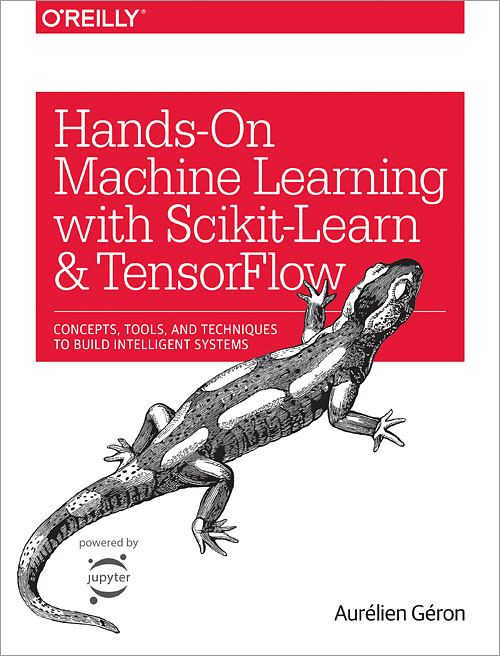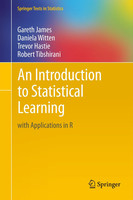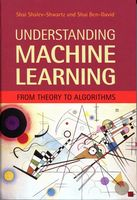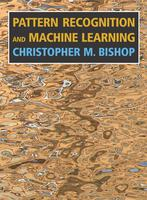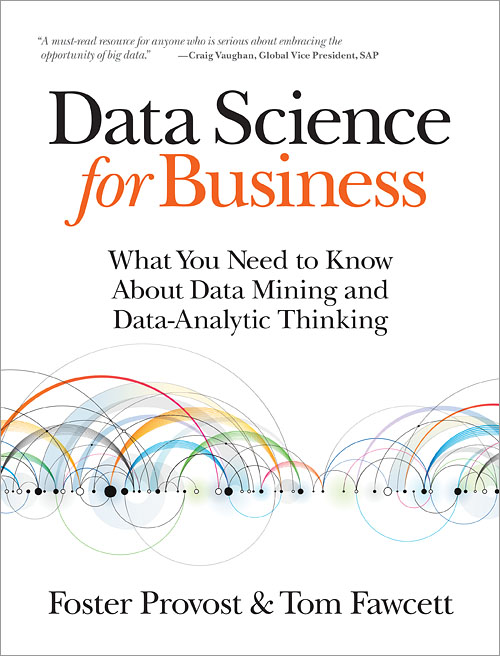Hands-On Machine Learning with Scikit-Learn and TensorFlow (Aurélien Géron)
This is a practical guide to machine learning that corresponds fairly well with the content and level of our course. While most of our homework is about coding ML from scratch with numpy, this book makes heavy use of scikit-learn and TensorFlow. We'll use the first two chapters of this book in the first two weeks of the course, when we cover "black-box machine learning." It'll also be a handy reference for your projects and beyond this course, when you'll want to make use of existing ML packages, rather than rolling your own.
The Elements of Statistical Learning (Hastie, Friedman, and Tibshirani)
This will be our main textbook for L1 and L2 regularization, trees, bagging, random forests, and boosting. It's written by three statisticians who invented many of the techniques discussed. There's an easier version of this book that covers many of the same topics, described below. (Available for free as a PDF.)
An Introduction to Statistical Learning (James, Witten, Hastie, and Tibshirani)
This book is written by two of the same authors as The Elements of Statistical Learning. It's much less intense mathematically, and it's good for a lighter introduction to the topics. Uses R as the language of instruction. (Available for free as a PDF.)
Understanding Machine Learning: From Theory to Algorithms (Shalev-Shwartz and Ben-David)
This is our primary reference for kernel methods and multiclass classification, and possibly more towards the end of the course. Covers a lot of theory that we don't go into, but it would be a good supplemental resource for a more theoretical course, such as Mohri's Foundations of Machine Learning course. (Available for free as a PDF.)
Pattern Recognition and Machine Learning (Christopher Bishop)
Our primary reference for probabilistic methods, including bayesian regression, latent variable models, and the EM algorithm. It's highly recommended, but unfortunately not free online.
Data Science for Business (Provost and Fawcett)
Ideally, this would be everybody's first book on machine learning. The intended audience is both the ML practitioner and the ML product manager. It's full of important core concepts and practical wisdom. The math is so minimal that it's perfect for reading on your phone, and I encourage you to read it in parallel to doing this class. Have your managers read it too.